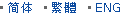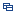| Recent Visit History | Join Audited Suppliers | Help | Region Guide
» » » » Nanjing Product List

211.

## Custom Made Glass Meniscus Lens Ar Coated[Nov 20, 2020]

Meniscus lenses have one concave and one convex surface. For this lens type, the two curvatures are chosen to minimize the spherical aberration at one laser wavelength, hence ...

212.

## Fused Silica Glass Focusing Lens[Nov 20, 2020]

Plano convex lenses are flat on one side and with a precision convex surface on another side. Plano convex lenses are used to focus, collect and collimate the light to one point. ...

213.

## Factory Glass Optics Meniscus Lens[Nov 20, 2020]

Meniscus lenses have one concave and one convex surface. For this lens type, the two curvatures are chosen to minimize the spherical aberration at one laser wavelength, hence ...

214.

## Bk7 Glass Optical Collimating Lens[Nov 20, 2020]

Plano convex lenses are flat on one side and with a precision convex surface on another side. Plano convex lenses are used to focus, collect and collimate the light to one point. ...

215.

## Bk7 Glass Optical Magnifying Lens[Nov 20, 2020]

Plano convex lenses are flat on one side and with a precision convex surface on another side. Plano convex lenses are used to focus, collect and collimate the light to one point. ...

216.

## Optical Glass N-Bk7 Positive Meniscus Lens[Nov 20, 2020]

Meniscus lenses have one concave and one convex surface. For this lens type, the two curvatures are chosen to minimize the spherical aberration at one laser wavelength, hence ...

217.

## Positive Lens Glass Lens Spherical Convex Lens[Nov 20, 2020]

Spherical lenses include plano convex lens, plano concave lens, double convex lens, double concave lens, meniscus lens, and achromatic lens. Double convex lenses have two outward ...

218.

## Manufacturer Optical Bk7 Glass Optics[Nov 20, 2020]

Double concave lenses have equal radius of curvature on both surfaces. The lenses are often used to expand light beams or to increase focal lengths in optical systems and devices, ...

219.

## Factory Bk7 Glass Cylindrical Lens[Nov 20, 2020]

A cylindrical lens is a lens which focuses light into a line instead of a point, as a spherical lens would. The curved surface or surfaces of a cylindrical lens are sections of a ...

220.

## Sapphire Glass Double Convex Spherical Lens[Nov 20, 2020]

Double convex lenses have two outward curved surfaces, normally the two surfaces have same radius of curvature, with finely polished. Bi convex lenses have positive focal length, ...

221.

## Optical Glass Meniscus Lens for Lighting[Nov 20, 2020]

Meniscus lenses have one concave and one convex surface. For this lens type, the two curvatures are chosen to minimize the spherical aberration at one laser wavelength, hence ...

222.

## Optical Glass Bk7 Diverging Lens[Nov 20, 2020]

Double concave lenses have equal radius of curvature on both surfaces. The lenses are often used to expand light beams or to increase focal lengths in optical systems and devices, ...

223.

## Bk7 Glass Lens-Fused Silica Glass Lens[Nov 20, 2020]

Bi concave lenses comprise of two inward equally curved surfaces. They are ideal for beam expansion, light projection and expanding the focal length of an optical system. Bi ...

224.

## Optical Component Optical Glass Lens Precision Optics Lens[Nov 20, 2020]

Meniscus lenses have one concave and one convex surface. For this lens type, the two curvatures are chosen to minimize the spherical aberration at one laser wavelength, hence ...

225.

## Optical Glass Bk7 Biconcave Lens[Nov 20, 2020]

Bi concave lenses comprise of two inward equally curved surfaces. They are ideal for beam expansion, light projection and expanding the focal length of an optical system. Bi ...

Hot Region Index: ABCDEFGHIJKLMNPQRSTUWXYZ